# 3D Printing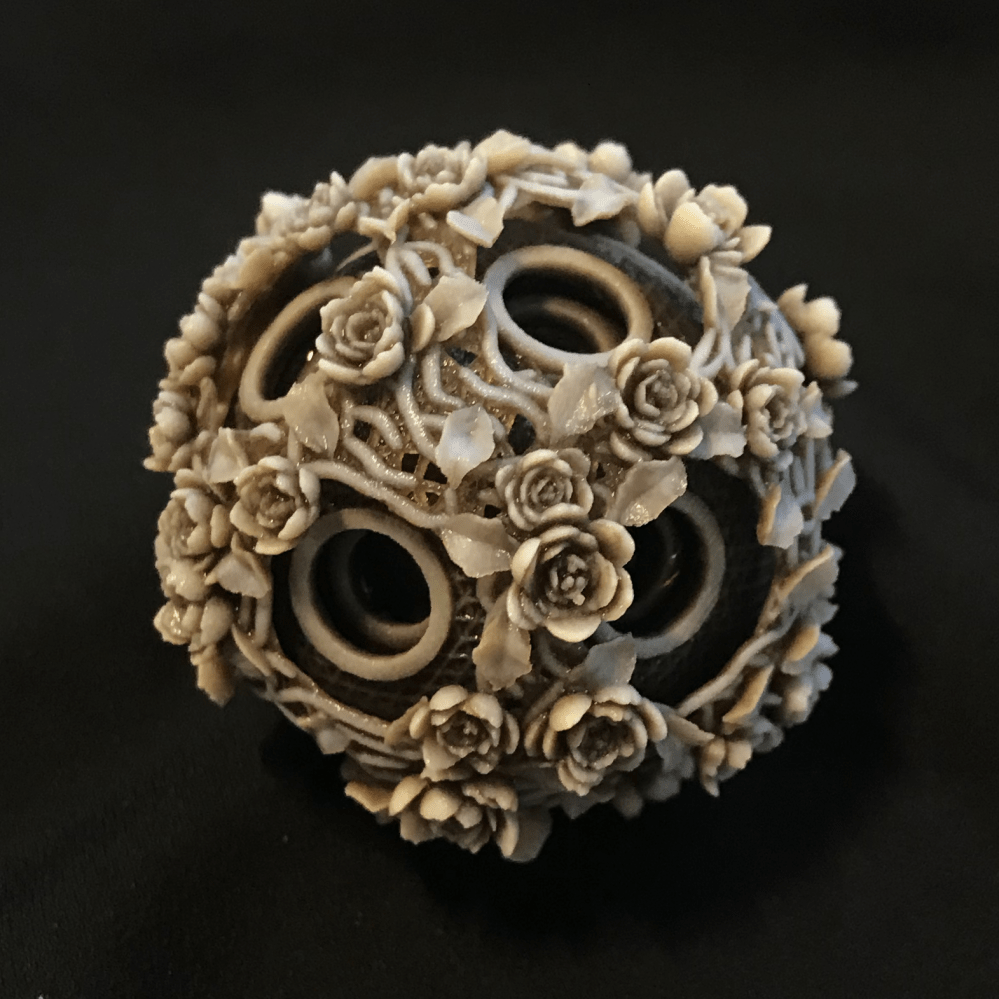This week we're asked to use 3D printers to make something that is otherwise difficult to produce with subtractive processes. Though it is the first time I ever use a 3D printer, I didn't have any difficulty resonating with that goal, since I had something complex in mind.

I was inspired by Chinese puzzle balls, which are spheres that recursively contain many layers of concentric spheres carved out out of a single piece of material. Though the mechanics is interesting, I'm more interested in reproducing the intricate reliefs on the spheres' surfaces, and how the holed patterns produce a rich visual effect when layered.

I wanted to generate the model procedurally with code, which is an interesting challenge for me, for though I have much experience generating 2D visuals with code, I have somewhat less with 3D ones. For one thing, it initially occurred to me that everything would need to be calculated in spherical coordinates (and the spheres have holes on them!). Oh no, non-euclidean math and CSG!To relieve the readers of any potential suspense: I managed to pull it off and you can read my full code below. It is written in around 1300 lines of JavaScript with no libraries used (no, not even three.js). It generates a STL file when run in the command-line, or you can also try the animated online demo here.

let LOAD_FRAME = false;
let IS_NODE = typeof require != 'undefined';

if (typeof globalThis != 'undefined'){
if (!globalThis.print_info) {globalThis.print_info = function(x){console.log(x)};}
}

let cos = Math.cos;
let sin = Math.sin;
let PI = Math.PI;

let jsr = 0x5EED;

function rand(){
jsr^=(jsr<<17);
jsr^=(jsr>>13);
jsr^=(jsr<<5);
return (jsr>>>0)/4294967295;
}

var PERLIN_YWRAPB = 4; var PERLIN_YWRAP = 1<<PERLIN_YWRAPB;
var PERLIN_ZWRAPB = 8; var PERLIN_ZWRAP = 1<<PERLIN_ZWRAPB;
var PERLIN_SIZE = 4095;
var perlin_octaves = 4;var perlin_amp_falloff = 0.5;
var scaled_cosine = function(i) {return 0.5*(1.0-Math.cos(i*PI));};
var perlin;
let noise = function(x,y,z) {
y = y || 0; z = z || 0;
if (perlin == null) {
perlin = new Array(PERLIN_SIZE + 1);
for (var i = 0; i < PERLIN_SIZE + 1; i++) {
perlin[i] = rand();
}
}
if (x<0) { x=-x; } if (y<0) { y=-y; } if (z<0) { z=-z; }
var xi=Math.floor(x), yi=Math.floor(y), zi=Math.floor(z);
var xf = x - xi; var yf = y - yi; var zf = z - zi;
var rxf, ryf;
var r=0; var ampl=0.5;
var n1,n2,n3;
for (var o=0; o<perlin_octaves; o++) {
var of=xi+(yi<<PERLIN_YWRAPB)+(zi<<PERLIN_ZWRAPB);
rxf = scaled_cosine(xf); ryf = scaled_cosine(yf);
n1  = perlin[of&PERLIN_SIZE];
n1 += rxf*(perlin[(of+1)&PERLIN_SIZE]-n1);
n2  = perlin[(of+PERLIN_YWRAP)&PERLIN_SIZE];
n2 += rxf*(perlin[(of+PERLIN_YWRAP+1)&PERLIN_SIZE]-n2);
n1 += ryf*(n2-n1);
of += PERLIN_ZWRAP;
n2  = perlin[of&PERLIN_SIZE];
n2 += rxf*(perlin[(of+1)&PERLIN_SIZE]-n2);
n3  = perlin[(of+PERLIN_YWRAP)&PERLIN_SIZE];
n3 += rxf*(perlin[(of+PERLIN_YWRAP+1)&PERLIN_SIZE]-n3);
n2 += ryf*(n3-n2);
n1 += scaled_cosine(zf)*(n2-n1);
r += n1*ampl;
ampl *= perlin_amp_falloff;
xi<<=1; xf*=2; yi<<=1; yf*=2; zi<<=1; zf*=2;
if (xf>=1.0) { xi++; xf--; }
if (yf>=1.0) { yi++; yf--; }
if (zf>=1.0) { zi++; zf--; }
}
return r;
};

function dist2(x0,y0,z0,x1,y1,z1){
let dx = x0-x1;
let dy = y0-y1;
let dz = z0-z1;
return dx*dx+dy*dy+dz*dz;
}

function dist(x0,y0,z0,x1,y1,z1){
return Math.sqrt(dist2(x0,y0,z0,x1,y1,z1));
}
function lerp(a,b,t){
return a*(1-t)+b*t;
}
function lerp3d(a,b,t){
return [
a*(1-t)+b*t,
a*(1-t)+b*t,
a*(1-t)+b*t,
]
}

return [x0+x1,y0+y1,z0+z1]
}
function v_sub(x0,y0,z0,x1,y1,z1){
return [x0-x1,y0-y1,z0-z1]
}

function v_scale(x0,y0,z0,s){
return [x0*s,y0*s,z0*s];
}

function v_mag(x,y,z){
return Math.sqrt(x*x+y*y+z*z)
}
function v_norm(x,y,z){
let l = v_mag(x,y,z);
return [x/l,y/l,z/l];
}

function v_cross(a1,a2,a3,b1,b2,b3){
return [(a2)*(b3)-(a3)*(b2),(a3)*(b1)-(a1)*(b3),(a1)*(b2)-(a2)*(b1)]
}
function v_dot(a1,a2,a3,b1,b2,b3){
return ((a1)*(b1)+(a2)*(b2)+(a3)*(b3));
}
function v_ang(ux,uy,uz,vx,vy,vz){
let d = v_dot(ux,uy,uz,vx,vy,vz);
let mu = v_mag(ux,uy,uz);
let mv = v_mag(vx,vy,vz);
return Math.acos(d/(mu*mv));
}
function m_ident(){
return [1,0,0,0, 0,1,0,0, 0,0,1,0, 0,0,0,1];
}
function m_rotx(a){
return [1,0,0,0, 0,cos(a),-sin(a),0, 0,sin(a),cos(a),0, 0,0,0,1];
}
function m_roty(a){
return [cos(a),0,sin(a),0, 0,1,0,0, -sin(a),0,cos(a),0, 0,0,0,1];
}
function m_rotz(a){
return [cos(a),-sin(a),0,0, sin(a),cos(a),0,0, 0,0,1,0, 0,0,0,1];
}
function m_trsl(x,y,z){
return [1,0,0,x, 0,1,0,y, 0,0,1,z, 0,0,0,1];
}
function m_scal(x,y,z){
return [x,0,0,0, 0,y,0,0, 0,0,z,0, 0,0,0,1];
}
function m_mult(A,B){
return [(A)*(B)+(A)*(B)+(A)*(B)+(A)*(B),(A)*(B)+(A)*(B)+(A)*(B)+(A)*(B),(A)*(B)+(A)*(B)+(A)*(B)+(A)*(B),(A)*(B)+(A)*(B)+(A)*(B)+(A)*(B),(A)*(B)+(A)*(B)+(A)*(B)+(A)*(B),(A)*(B)+(A)*(B)+(A)*(B)+(A)*(B),(A)*(B)+(A)*(B)+(A)*(B)+(A)*(B),(A)*(B)+(A)*(B)+(A)*(B)+(A)*(B),(A)*(B)+(A)*(B)+(A)*(B)+(A)*(B),(A)*(B)+(A)*(B)+(A)*(B)+(A)*(B),(A)*(B)+(A)*(B)+(A)*(B)+(A)*(B),(A)*(B)+(A)*(B)+(A)*(B)+(A)*(B),(A)*(B)+(A)*(B)+(A)*(B)+(A)*(B),(A)*(B)+(A)*(B)+(A)*(B)+(A)*(B),(A)*(B)+(A)*(B)+(A)*(B)+(A)*(B),(A)*(B)+(A)*(B)+(A)*(B)+(A)*(B)];
}
function m_tfrm(A,v){
return [((A)*(v)+(A)*(v)+(A)*(v)+(A))/((A)*(v)+(A)*(v)+(A)*(v)+(A)),((A)*(v)+(A)*(v)+(A)*(v)+(A))/((A)*(v)+(A)*(v)+(A)*(v)+(A)),((A)*(v)+(A)*(v)+(A)*(v)+(A))/((A)*(v)+(A)*(v)+(A)*(v)+(A))];
}
function m_rot_axis(ux,uy,uz,th){
let costh = cos(th);
let sinth = sin(th);
return [
costh+ux*ux*costh, ux*uy*(1-costh)-uz*sinth, ux*uz*(1-costh)+uy*sinth, 0,
uy*ux*(1-costh)+uz*sinth, costh+uy*uy*(1-costh), uy*uz*(1-costh)-ux*sinth, 0,
uz*ux*(1-costh)-uy*sinth, uz*uy*(1-costh)+ux*sinth, costh+uz*uz*(1-costh), 0,
0,0,0,1
];
}

function rot_euler(x,y,z,rx,ry,rz){
let M = m_mult(m_rotz(rz),m_mult(m_roty(ry),m_rotx(rx)));
return m_tfrm(M,[x,y,z]);
}

function trfm_faces(A,ff){
for (let i = 0; i < ff.length; i++){
for (let j = 0; j < ff[i].length; j++){
ff[i][j] = m_tfrm(A,ff[i][j]);
}
}
return ff;
}
function clone(ff){
return JSON.parse(JSON.stringify(ff));
}

function icosphere(order = 0){
//copied from https://observablehq.com/@mourner/fast-icosphere-mesh by Vladimir Agafonkin

// set up a 20-triangle icosahedron
const f = (1 + 5 ** 0.5) / 2;
const T = 4 ** order;

const vertices = new Float32Array((10 * T + 2) * 3);
vertices.set(Float32Array.of(
-1, f, 0, 1, f, 0, -1, -f, 0, 1, -f, 0,
0, -1, f, 0, 1, f, 0, -1, -f, 0, 1, -f,
f, 0, -1, f, 0, 1, -f, 0, -1, -f, 0, 1));
let triangles = Uint32Array.of(
0, 11, 5, 0, 5, 1, 0, 1, 7, 0, 7, 10, 0, 10, 11,
11, 10, 2, 5, 11, 4, 1, 5, 9, 7, 1, 8, 10, 7, 6,
3, 9, 4, 3, 4, 2, 3, 2, 6, 3, 6, 8, 3, 8, 9,
9, 8, 1, 4, 9, 5, 2, 4, 11, 6, 2, 10, 8, 6, 7);

let v = 12;
const midCache = order ? new Map() : null; // midpoint vertices cache to avoid duplicating shared vertices

const key = Math.floor((a + b) * (a + b + 1) / 2) + Math.min(a, b); // Cantor's pairing function
let i = midCache.get(key);
if (i !== undefined) { midCache.delete(key); return i; }
midCache.set(key, v);
for (let k = 0; k < 3; k++) vertices[3 * v + k] = (vertices[3 * a + k] + vertices[3 * b + k]) / 2;
i = v++;
return i;
}

let trianglesPrev = triangles;
for (let i = 0; i < order; i++) {
// subdivide each triangle into 4 triangles
triangles = new Uint32Array(trianglesPrev.length * 4);
for (let k = 0; k < trianglesPrev.length; k += 3) {
const v1 = trianglesPrev[k + 0];
const v2 = trianglesPrev[k + 1];
const v3 = trianglesPrev[k + 2];
let t = k * 4;
triangles[t++] = v1; triangles[t++] = a; triangles[t++] = c;
triangles[t++] = v2; triangles[t++] = b; triangles[t++] = a;
triangles[t++] = v3; triangles[t++] = c; triangles[t++] = b;
triangles[t++] = a;  triangles[t++] = b; triangles[t++] = c;
}
trianglesPrev = triangles;
}
// normalize vertices
for (let i = 0; i < vertices.length; i += 3) {
const m = 1 / Math.hypot(vertices[i + 0], vertices[i + 1], vertices[i + 2]);
vertices[i + 0] *= m;
vertices[i + 1] *= m;
vertices[i + 2] *= m;
}
return {vertices, triangles};
}

function mark_vertex(x,y,z,s){
let d = s/2;
let fa = [
[[-d,-d,-d],[+d,+d,-d],[+d,-d,-d]],
[[-d,-d,-d],[-d,+d,-d],[+d,+d,-d]],
[[-d,-d,+d],[+d,-d,+d],[+d,+d,+d]],
[[-d,-d,+d],[+d,+d,+d],[-d,+d,+d]],
]
let fb = trfm_faces(m_rotx(PI/2),clone(fa));
let fc = trfm_faces(m_roty(PI/2),clone(fa));
let ff = fa.concat(fb).concat(fc);
return trfm_faces(m_trsl(x,y,z),ff);
}

function look_at( eye, target, up ){
//https://github.com/mrdoob/three.js/blob/342946c8392639028da439b6dc0597e58209c696/src/math/Matrix4.js#L293
// let z = [eye-target,eye-target,eye-target];
let z = [-eye+target,-eye+target,-eye+target];
if (v_mag(...z) == 0){
z = 1;
}
z = v_norm(...z);

let x = v_cross(...up,...z);
if (v_mag(...x) == 0){
if (Math.abs(up)==1){
z += 0.0001;
}else{
z += 0.0001;
}
z = v_norm(...z);
x = v_cross(...up,...z);
}
x = v_norm(...x);
let y = v_cross(...z,...x);
let te = m_ident();
te[ 0 ] = x; te[ 1 ] = y; te[ 2 ] = z;
te[ 4 ] = x; te[ 5 ] = y; te[ 6 ] = z;
te[ 8 ] = x; te[ 9 ] = y; te[ 10 ] =z;
return te;
}

// let M = look_at([0,0,0],[0,1,2],[0,1,0]);
// console.log(m_tfrm(M,[0,0,1]))

function mark_edge(x0,y0,z0,x1,y1,z1,s){
let l = dist(x0,y0,z0,x1,y1,z1);
let d = s/2;
let ff = mark_vertex(0,0,0,s);
ff = trfm_faces(m_trsl(0,0,l/2),
trfm_faces(m_scal(1,1,l/s),ff));

let M = look_at([x0,y0,z0],[x1,y1,z1],[0,1,0]);
// return ff;
return trfm_faces(m_trsl(x0,y0,z0),trfm_faces(M,ff));

}

// function to_stl(faces){
//   let o = solid\n;

//   for (let i = 0; i < faces.length; i++){
//     let [a,b,c] = faces[i];
//     o += 
//   facet normal 0 0 0
//     outer loop
//       vertex ${a}${a} ${a} // vertex${b} ${b}${b}
//       vertex ${c}${c} ${c} // endloop // endfacet // ; // } // o += endsolid; // return o; // } function to_stl(faces){ print_info(writing stl (ascii)...); let o = ['solid']; for (let i = 0; i < faces.length; i++){ let [a,b,c] = faces[i]; o.push( facet normal 0 0 0 outer loop vertex${a} ${a}${a}
vertex ${b}${b} ${b} vertex${c} ${c}${c}
endloop
endfacet
);
}
o.push('endsolid');
return o.join('\n');
}

function to_stl_bin(faces){
let nb = 84+faces.length*50;
print_info(writing stl (binary)... estimated ${~~(nb/1048576)} MB); let o = new Uint8Array(nb); let a = new ArrayBuffer(4); let b = new Uint32Array(a); b = faces.length; o.set(new Uint8Array(a),80); for (let i = 0; i < faces.length; i++){ let d = [ faces[i],faces[i],faces[i], faces[i],faces[i],faces[i], faces[i],faces[i],faces[i], ] let a = new ArrayBuffer(36); let b = new Float32Array(a); d.map((x,j)=>b[j]=x); o.set(new Uint8Array(a),84+i*50+12); } return o; } function uni_sphere_sampler(){ let Xi1 = rand(); let Xi2 = rand(); let theta = Math.acos(Xi1); let phi = 2*PI*Xi2; let xs = sin(theta)*cos(phi); let ys = sin(theta)*sin(phi); let zs = cos(theta); if (rand() < 0.5){ zs = -zs; } return [xs,ys,zs]; } function sphere_samples(N=1000,K=10){ let samples = []; for (let i = 0; i < N; i++){ if (i%100==0)print_info(generating anchor${i} / ${N}); let dmin = -Infinity; let np; for (let j = 0; j < K; j++){ let p = uni_sphere_sampler(); let d = Infinity; for (let k = 0; k < samples.length; k++){ d = Math.min(d,dist2(...p,...samples[k])); } if (d > dmin){ dmin = d; np = p; } } samples.push(np); } return samples; } let DRILL_PTS = [ [-1,0,0], [1,0,0], [0,-1,0], [0,1,0], [0,0,-1], [0,0,1], rot_euler(0,0,1, 0.61548,PI*1/4,0), rot_euler(0,0,1, 0.61548,PI*3/4,0), rot_euler(0,0,1, 0.61548,PI*5/4,0), rot_euler(0,0,1, 0.61548,PI*7/4,0), rot_euler(0,0,1,-0.61548,PI*1/4,0), rot_euler(0,0,1,-0.61548,PI*3/4,0), rot_euler(0,0,1,-0.61548,PI*5/4,0), rot_euler(0,0,1,-0.61548,PI*7/4,0), ]; function drill_sphere(samples,s){ let pts = DRILL_PTS; for (let i = samples.length-1; i >= 0; i--){ for (let j = 0; j < pts.length; j++){ if (dist2(...samples[i],...pts[j]) < s*s){ samples.splice(i,1); break; } } } } function make_neighbors_d(samples,maxd){ let dd = maxd*maxd; let N = new Array(samples.length).fill(0).map(_=>[]); for (let i = 0; i < samples.length; i++){ let a = samples[i]; for (let j = 0; j < i; j++){ let b = samples[j]; if (dist2(...a,...b) < dd){ N[i].push(j); N[j].push(i); } } } return N; } function make_neighbors_n(samples,num){ let N = []; for (let i = 0; i < samples.length; i++){ if (i%100 == 0) print_info(building neighbors${i} / ${samples.length}); let dists = samples.map(q=>(dist2(...samples[i],...q))); let nbrs = samples.slice().map((q,i)=>[i,dists[i]]).sort((a,b)=>[a-b]).slice(1,num+1).map(x=>x); N.push(nbrs); } return N; } function run_vine(samples,nbrs,visits){ let p0; do{ p0 = ~~(rand()*samples.length); }while (visits[p0]); let ps = [p0]; visits[p0]++; let lvls = ; for (let i = 0; i < 100; i++){ let idx1 = ps[ps.length-1] let [x1,y1,z1] = samples[idx1]; let cands = nbrs[idx1]; let x0,y0,z0; if (ps.length >= 2){ ;[x0,y0,z0] = samples[ps[ps.length-2]]; } let da = Infinity; let q; for (let j = 0; j < cands.length; j++){ let a = 1; if (ps.length >= 2){ let [x2,y2,z2] = samples[cands[j]]; let u = [x1-x0,y1-y0,z1-z0]; let v = [x2-x1,y2-y1,z2-z1]; a = v_ang(...u,...v); } a *= (visits[cands[j]]+1)*0.5+0.5; if (a < da){ da = a; q = cands[j]; } } ps.push(q); visits[q] ++; if (visits[q] >= 2){ lvls.push(0); break; }else{ lvls.push(visits[q]-1); } } let qs = []; for (let i = 0 ; i< ps.length; i++){ let p = samples[ps[i]]; let n = v_norm(...samples[ps[i]]); p += n * 0.02 * lvls[i]; p += n * 0.02 * lvls[i]; p += n * 0.02 * lvls[i]; qs.push(p); } return qs; } function pt_seg_dist(p, p0, p1) { // https://stackoverflow.com/a/6853926 let x = p; let y = p; let x1 = p0; let y1 = p0; let x2 = p1; let y2 = p1; let A = x - x1; let B = y - y1; let C = x2 - x1; let D = y2 - y1; let dot = A*C+B*D; let len_sq = C*C+D*D; let param = -1; if (len_sq != 0) { param = dot / len_sq; } let xx; let yy; if (param < 0) { xx = x1; yy = y1; }else if (param > 1) { xx = x2; yy = y2; }else { xx = x1 + param*C; yy = y1 + param*D; } let dx = x - xx; let dy = y - yy; return Math.sqrt(dx*dx+dy*dy); } function approx_poly_dp(polyline, epsilon){ if (polyline.length <= 2){ return polyline; } let dmax = 0; let argmax = -1; for (let i = 1; i < polyline.length-1; i++){ let d = pt_seg_dist(polyline[i] , polyline , polyline[polyline.length-1] ); if (d > dmax){ dmax = d; argmax = i; } } let ret = []; if (dmax > epsilon){ let L = approx_poly_dp(polyline.slice(0,argmax+1),epsilon); let R = approx_poly_dp(polyline.slice(argmax,polyline.length),epsilon); ret = ret.concat(L.slice(0,L.length-1)).concat(R); }else{ ret.push(polyline.slice()); ret.push(polyline[polyline.length-1].slice()); } return ret; } function smooth_vine(vine){ let ps = catmull(vine,10,0.5).flat(); ps = approx_poly_dp(ps,0.0005); return ps; } function draw_vine(vine,wr=0.025){ let nr = 10; let rings = []; for (let i = 0; i < vine.length; i++){ let t = (i/(vine.length-1)); let ww = wr* (Math.pow(Math.sin(t*PI),1)*0.3+0.7); // let ww = wr; let ring = []; let a = vine[i]; let d; if (i < vine.length-1){ let b = vine[i+1]; d = [ b-a, b-a, b-a, ]; }else{ let b = vine[i-1]; d = [ a-b, a-b, a-b, ] } let n = v_norm(...a); // d = v_scale(...v_norm(...d),0.1); d = v_norm(...d); let rat = 1.5; for (let k = 0; k < 2; k++){ for (let j = 0; j < nr; j++){ let x = -((j/nr)*2-1)/rat; let y = Math.sqrt((1-rat*rat*x*x)); let th = Math.atan2(y,x)+PI*k; let M = m_rot_axis(...d,th); let v = m_tfrm(M,n); let w = ww * Math.sqrt(x*x+y*y); v = v_scale(...v,w); ring.push(v_add(...a,...v)); } } rings.push(ring); } let ff = []; let pr = wr*0.8; { let a = vine; let b = vine; let d = v_scale(...v_norm(...v_sub(...a,...b)),pr); let c = v_add(...a,...d); let r0 = rings; for (let j = 0; j < r0.length; j++){ let k = (j+1)%r0.length; ff.push([c,r0[k],r0[j]]); } } for (let i = 0; i < rings.length-1; i++){ let r0 = rings[i]; let r1 = rings[i+1]; for (let j = 0; j < r0.length; j++){ let k = (j+1)%r0.length; ff.push([r0[j],r0[k],r1[k]]); ff.push([r0[j],r1[k],r1[j]]); } } { let a = vine[vine.length-1]; let b = vine[vine.length-2]; let d = v_scale(...v_norm(...v_sub(...a,...b)),pr); let c = v_add(...a,...d); let r0 = rings[rings.length-1]; for (let j = 0; j < r0.length; j++){ let k = (j+1)%r0.length; ff.push([c,r0[k],r0[j]]); } } return ff; } // function make_leaf(w,h,d){ // let stm0 = []; // let stm1 = []; // let riml = []; // let rimr = []; // let n = 20; // for (let i = 0; i < n; i++){ // let t = i/(n-1); // let x = sin(PI*Math.pow( (1-t),1.5)); // let dd = x*d*1.5; // let b = Math.sin(t*PI)*d; // stm0.push([0, 0+b,t*h]); // stm1.push([0,-dd+b,t*h]); // riml.push([-x*w,dd+b,t*h]); // rimr.push([x*w, dd+b,t*h]); // } // let ff = []; // // for (let i = 0; i < stem.length-1; i++){ // // ff.push([riml[i], riml[i+1], rimr[i+1] ]); // // ff.push([riml[i], rimr[i+1], rimr[i] ]); // // } // for (let i = 0; i < stm0.length-1; i++){ // ff.push([riml[i], riml[i+1], stm0[i+1] ]); // ff.push([riml[i], stm0[i+1], stm0[i] ]); // ff.push([stm0[i], stm0[i+1], rimr[i+1] ]); // ff.push([stm0[i], rimr[i+1], rimr[i] ]); // ff.push([riml[i+1], riml[i], stm1[i+1] ]); // ff.push([stm1[i+1], riml[i], stm1[i] ]); // ff.push([stm1[i+1], stm1[i], rimr[i+1] ]); // ff.push([rimr[i+1], stm1[i], rimr[i] ]); // } // return ff; // } function make_leaf(w,h,d){ let stm0 = []; let stm1 = []; let riml = []; let rimr = []; let n = 24; let m = 12; let sd = rand(); function fun(t){ if (t > 0.1){ t = (t-0.1)/0.9 return sin(PI*Math.pow( (1-t),1.2)); }else{ return 0.1; } } for (let i = 0; i < n; i++){ let t = i/(n-1); let x = fun(t); let x0 = x*(noise(sd,t*10)*0.8+0.6); let x1 = x*(noise(sd,t*10,PI)*0.8+0.6); let dd = x*d*3; let b = Math.sin(t*PI)*d; stm0.push([0, 0+b,t*h]); stm1.push([0,-dd+b,t*h]); riml.push([-x0*w,dd+b,t*h]); rimr.push([x1*w, dd+b,t*h]); } let s0 = new Array(n).fill(0).map(_=>new Array(m*4).fill(0)); let cr = 0.2; for (let i = 0; i < n; i++){ let a = riml[i]; let b = stm0[i]; for (let j = 0; j < m; j++){ let t = cr+(1-cr)*(j/m); let c = lerp3d(a,b,t); s0[i][j] = c; } a = stm0[i]; b = rimr[i]; for (let j = 0; j < m; j++){ let t = (j/(m-1))*(1-cr); let c = lerp3d(a,b,t); s0[i][m+j] = c; } a = rimr[i]; b = stm1[i]; for (let j = 0; j < m; j++){ let t = cr+(1-cr)*(j/m); let c = lerp3d(a,b,t); s0[i][m*2+j] = c; } a = stm1[i]; b = riml[i]; for (let j = 0; j < m; j++){ let t = (j/(m-1))*(1-cr); let c = lerp3d(a,b,t); s0[i][m*3+j] = c; } } for (let i = 0; i < s0.length; i++){ for (let j = 0; j < s0[i].length; j++){ let p = s0[i][j]; let n = noise(sd,p*2,p*2); s0[i][j] += n*0.5-0.25; } } let dy = s0[m]; let ang = Math.atan2(s0[s0.length-1][m]-dy,s0[s0.length-1][m]); let rm = m_rotx(ang); for (let i = 0; i < s0.length; i++){ for (let j = 0; j < s0[i].length; j++){ s0[i][j] -= dy; s0[i][j] = m_tfrm(rm,s0[i][j]); } } let ff = []; // for (let i = 0; i < stem.length-1; i++){ // ff.push([riml[i], riml[i+1], rimr[i+1] ]); // ff.push([riml[i], rimr[i+1], rimr[i] ]); // } for (let i = 0; i < s0.length-1; i++){ let r0 = s0[i]; let r1 = s0[i+1]; for (let j = 0; j < r0.length; j++){ let k = (j+1) % r0.length; ff.push([r0[k],r0[j],r1[k]]); ff.push([r1[k],r0[j],r1[j]]); } } return ff; } // function leaf_vine(vine){ // let ff = []; // let freq = 200; // for (let i = 0; i < vine.length-1; i++){ // if (i != 0){ // continue; // } // let lr = ((i/freq)%2)*2-1; // let leaf = make_leaf(0.1,0.3,0.02); // let a = vine[i]; // let b = vine[i+1]; // let d = v_sub(...b,...a); // let n = v_norm(...a); // let M = m_rot_axis(...n,PI/4*lr); // let v = m_tfrm(M,d); // let A = look_at([0,0,0],v,n); // A = m_mult(m_trsl(...a),A); // leaf = trfm_faces(A,leaf); // ff.push(...leaf); // } // return ff; // } function leaf_samples(samples,nbrs,picked){ let ff = []; for (let i = 0; i < samples.length; i++){ let lr = [-1,1][~~(rand()*2)] let a = samples[i]; let b = samples[nbrs[i]]; let d = v_sub(...b,...a); let n = v_norm(...a); let M = m_rot_axis(...n,PI/8*lr); let v = m_tfrm(M,d); let c = v_add(...a,...v_scale(...v_norm(...v),0.15)); // console.log(a,v,c); let ok = true; for (let j = 0; j < picked.length; j++){ if (dist(...picked[j],...c)<0.25){ ok = false; break; } } if (!ok) continue; print_info(generating leaves${i} / ${samples.length}); let leaf = make_leaf(0.1,0.3,0.02); let aa = v_add(...a,...v_scale(...n,0.008)); let A = look_at([0,0,0],v,n); A = m_mult(m_trsl(...aa),A); leaf = trfm_faces(A,leaf); ff.push(...leaf); picked.push(c); } return ff; } function flower_samples(samples,nbrs,picked){ let ff = []; for (let i = 0; i < samples.length; i++){ let s= rand()*0.4+0.6; let a = samples[i]; let ok = true; for (let j = 0; j < picked.length; j++){ if (dist2(...picked[j],...a)<0.2**2){ ok = false; break; } } if (!ok) continue; print_info(generating flowers${i} / ${samples.length}); let flower = trfm_faces(m_scal(0.1*s,0.1*s,0.1*s),make_flower()); let b = samples[nbrs[i]]; let d = v_sub(...b,...a); let n = v_norm(...a); let aa = v_add(...a,...v_scale(...n,0.008)); let A = look_at([0,0,0],d,n); A = m_mult(m_trsl(...aa),A); flower = trfm_faces(A,flower); ff.push(...flower); picked.push(a); } return ff; } function petal(){ let sd = rand(); let n = 12; let m = 16; function fun0(t){ return Math.sqrt(1-(t-1)**2); } function fun1(t){ return Math.sqrt(1-(2*t-1)**2)*(noise(sd,t*5)*0.8+0.2); } function fun2(t){ return Math.sqrt(1-(t-1)**2); } function fun3(t){ return Math.sqrt(1-(2*t-1)**2); } function fun4(t){ return Math.sqrt(1-(2*t-1)**2); } let sss = []; for (let i = 0; i < n; i++){ let ss = []; let t = i/(n-1); for (let j = 0; j < m; j++){ let s = j/(m); let thk = fun3(s)*fun3(t)*0.2; let x = lerp(-fun0(t),fun0(t),s); let y = t*(1+fun1(s))+thk; let z = fun2(t)-thk+fun4(s)*0.5; ss.push([x,y,z]); } for (let j = 0; j < m; j++){ let s = j/(m); let thk = fun3(s)*fun3(t)*0.2; let x = lerp(fun0(t),-fun0(t),s); let y = t*(1+fun1(1-s))-thk; let z = fun2(t)+thk+fun4(1-s)*0.5; ss.push([x,y,z]); } sss.push(ss); } let ff = []; for (let i = 0; i < sss.length-1; i++){ let r0 = sss[i]; let r1 = sss[i+1]; for (let j = 0; j < r0.length; j++){ let k = (j+1) % r0.length; ff.push([r0[k],r0[j],r1[k]]); ff.push([r1[k],r0[j],r1[j]]); } } return ff; } function make_flower(){ let n = 6; let m = 4; let ff = []; for (let k = 0; k < m; k++){ let a0 = PI*2*rand(); for (let i = 0; i < n; i++){ let t = i/n; let a = t*PI*2+a0; let M = m_mult(m_trsl(0,0,0.2-k*0.1),m_scal(0.8-k*0.1,1-k*0.1,1-k*0.2)); M = m_mult(m_rotx(rand()*(0.64-k*0.05)-0.1),M); M = m_mult(m_roty(a),M); let pet = petal(); ff.push(...trfm_faces(M,pet)); } } ff.push(...trfm_faces(m_scal(0.5,0.5,0.5),icosphere_faces(1))); let stamen = trfm_faces(m_scal(0.15,0.15,0.15),icosphere_faces(0)); for (let i = 0; i < 8; i++){ let p = [rand()-0.5,0.5+rand()*0.8,rand()-0.5]; ff.push(...trfm_faces(m_trsl(...p),clone(stamen))); ff.push(...mark_edge(...p,0,0,0,0.1)); } return ff; } function catmull(positions,num,alpha){ const EPSILON = 0.001; function get_t(t, p0, p1, alpha){ let a = Math.pow((p1-p0), 2.0) + Math.pow((p1-p0), 2.0) + Math.pow((p1-p0),2.0); let b = Math.pow(a, alpha * 0.5); return (b + t); } function spline(p0, p1, p2, p3, num, alpha){ //https://en.wikipedia.org/wiki/Centripetal_Catmull-Rom_spline let pts = []; if (p0 == p1 && p0 == p1 && p0 == p1) { p0 += EPSILON; } if (p1 == p2 && p1 == p2 && p1 == p2) { p1 += EPSILON; } if (p2 == p3 && p2 == p3 && p2 == p3) { p2 += EPSILON; } let t0 = 0.0; let t1 = get_t(t0, p0, p1,alpha); let t2 = get_t(t1, p1, p2,alpha); let t3 = get_t(t2, p2, p3,alpha); for (let t=t1; t<t2; t+=((t2-t1)/num)){ let A1 = v_add(...v_scale(...p0,(t1-t)/(t1-t0)) , ...v_scale(...p1,(t-t0)/(t1-t0)) ); let A2 = v_add(...v_scale(...p1,(t2-t)/(t2-t1)) , ...v_scale(...p2,(t-t1)/(t2-t1)) ); let A3 = v_add(...v_scale(...p2,(t3-t)/(t3-t2)) , ...v_scale(...p3,(t-t2)/(t3-t2)) ); let B1 = v_add(...v_scale(...A1,(t2-t)/(t2-t0)) , ...v_scale(...A2,(t-t0)/(t2-t0)) ); let B2 = v_add(...v_scale(...A2,(t3-t)/(t3-t1)) , ...v_scale(...A3,(t-t1)/(t3-t1)) ); let C = v_add(...v_scale(...B1,(t2-t)/(t2-t1)) , ...v_scale(...B2,(t-t1)/(t2-t1)) ); // console.log(p0,p1,p2,t0,t1,t2,A1,A2,A3,B1,B2,C) pts.push(C); } pts.push(p2.slice()); return pts; } let curves = []; for (let i = 0; i < positions.length-1; i++){ let p0 = positions[Math.max(i-1,0)]; let p1 = positions[i]; let p2 = positions[i+1]; let p3 = positions[Math.min(i+2,positions.length-1)]; let pts = spline(p0.slice(),p1.slice(),p2.slice(),p3.slice(),num,alpha); curves.push(pts); } return curves; } function icosphere_faces(order=0){ let {vertices,triangles} = icosphere(order); let ff = []; for (let i = 0; i < triangles.length; i+=3){ let a = triangles[i]; let b = triangles[i+1]; let c = triangles[i+2] ff.push([ [vertices[a*3],vertices[a*3+1],vertices[a*3+2]], [vertices[b*3],vertices[b*3+1],vertices[b*3+2]], [vertices[c*3],vertices[c*3+1],vertices[c*3+2]], ]); } return ff; } function make_stick(a,b){ let ww = 0.018; let pr = ww/2; let ff = [] let d = [ b-a, b-a, b-a, ]; let n = v_norm(...a); d = v_norm(...d); let nr = 4; let rat = 0.5; let r0 = []; let r1 = []; for (let k = 0; k < 2; k++){ for (let j = 0; j < nr; j++){ let x = -((j/nr)*2-1)/rat; let y = Math.sqrt((1-rat*rat*x*x)); let th = Math.atan2(y,x)+PI*k; let M = m_rot_axis(...d,th); let v = m_tfrm(M,n); let w = ww * Math.sqrt(x*x+y*y); v = v_scale(...v,w); r0.push(v_add(...a,...v)); r1.push(v_add(...b,...v)); } } { let d = v_scale(...v_norm(...v_sub(...a,...b)),pr); let c = v_add(...a,...d); for (let j = 0; j < r0.length; j++){ let k = (j+1)%r0.length; ff.push([c,r0[k],r0[j]]); } } for (let j = 0; j < r0.length; j++){ let k = (j+1)%r0.length; ff.push([r0[j],r0[k],r1[k]]); ff.push([r0[j],r1[k],r1[j]]); } { let d = v_scale(...v_norm(...v_sub(...b,...a)),pr); let c = v_add(...b,...d); for (let j = 0; j < r1.length; j++){ let k = (j+1)%r1.length; ff.push([c,r1[k],r1[j]]); } } return ff; } function icosphere_edges(order=1){ let {vertices,triangles} = icosphere(order); let edges = []; for (let i = 0; i < triangles.length; i+=3){ let a = triangles[i]; let b = triangles[i+1]; let c = triangles[i+2]; let e0 = a+','+b; let e1 = b+','+c; let e2 = c+','+a; let e3 = b+','+a; let e4 = c+','+b; let e5 = a+','+c; if (!edges.includes(e3)){ edges.push(e0); } if (!edges.includes(e4)){ edges.push(e1); } if (!edges.includes(e5)){ edges.push(e2); } } for (let i = 0; i < edges.length; i++){ let [a,b] = edges[i].split(','); edges[i] = [ vertices.slice(a*3,a*3+3), vertices.slice(b*3,b*3+3), ] } return edges; } function make_drilled_ico(r,s=0.25){ let edges = icosphere_edges(4); let pts = DRILL_PTS; for (let i = edges.length-1; i >= 0; i--){ for (let j = 0; j < pts.length; j++){ if (dist2(...edges[i],...pts[j]) < s*s){ edges.splice(i,1); break; } if (dist2(...edges[i],...pts[j]) < s*s){ edges.splice(i,1); break; } } } let ff = []; for (let i = 0; i < edges.length; i++){ ff.push(...make_stick(v_scale(...edges[i],r), v_scale(...edges[i],r))); } return ff; } function make_ring(rad0,rad1,thk){ let n = 20; let m = 12; let s0 = []; let s1 = []; let s2 = []; let s3 = []; for (let i = 0; i < n; i++){ let a = (i/n)*PI*2; s0.push([cos(a)*rad0,sin(a)*rad0,-thk]); s1.push([cos(a)*rad1,sin(a)*rad1,-thk]); s2.push([cos(a)*rad0,sin(a)*rad0,thk]); s3.push([cos(a)*rad1,sin(a)*rad1,thk]); } let ff = []; for (let i = 0; i < s0.length; i++){ let j = (i+1)%s0.length ff.push([s0[i],s1[j],s0[j]]); ff.push([s0[i],s1[i],s1[j]]); ff.push([s2[i],s2[j],s3[j]]); ff.push([s2[i],s3[j],s3[i]]); ff.push([s0[i],s0[j],s2[j]]); ff.push([s0[i],s2[j],s2[i]]); ff.push([s1[i],s3[j],s1[j]]); ff.push([s1[i],s3[i],s3[j]]); } // let stamen = trfm_faces(m_scal(0.015,0.015,0.015),icosphere_faces(0)); // for (let i = 0; i < m; i++){ // let a = (i/m)*PI*2; // let p = [ // cos(a)*(rad0+rad1)/2, // sin(a)*(rad0+rad1)/2, // thk, // ] // ff.push(...trfm_faces(m_trsl(...p),clone(stamen))); // } return ff; } function make_inner_ico(r){ let ff = []; let ico = make_drilled_ico(r); for (let i = 0; i < ico.length; i++){ ff.push(ico[i]); } let rin = make_ring(0.3*r,0.3*r-0.06,0.03); rin = trfm_faces(m_trsl(0,0,r),rin); let pts = DRILL_PTS; for (let i = 0; i < pts.length; i++){ let M = look_at([0,0,0],pts[i],[0,1,0]); ff.push(...trfm_faces(M,clone(rin))); } return ff; } function sphere_wires(samples,nbrs){ let faces = []; let done = new Array(samples.length).fill(0).map(_=>({})); for (let i = 0; i < samples.length; i++){ let d = v_norm(...samples[i]); let p = m_tfrm(m_trsl(...v_scale(...d,-0.015)),samples[i]); let ns = nbrs[i]; for (let j = 0; j < ns.length; j++){ if (done[i][ns[j]]){ continue; } done[ns[j]][i] = true; let e = v_norm(...samples[ns[j]]); let q = m_tfrm(m_trsl(...v_scale(...e,-0.015)),samples[ns[j]]); faces.push(...mark_edge(...p,...q,0.02)); } } return faces; } function make_outer_shell(samples,nbrs){ let faces = []; // for (let i = 0; i < samples.length; i++){ // faces.push(...mark_vertex(...samples[i],0.01)) // } faces.push(...sphere_wires(samples,nbrs)); let visits = new Array(samples.length).fill(0); let n = 0; while(visits.includes(0)){ n++; if (n%50==0) print_info(generating vine${n});

let vine = smooth_vine(run_vine(samples,nbrs,visits));
let ff = draw_vine(vine);
faces.push(...ff);
// ff = leaf_vine(vine);
// for (let i = 0; i < vine.length-1; i++){
//   faces.push(...mark_edge(...vine[i],...vine[i+1],0.02));
// }
// break;
}
let picked = [];
let leaves = leaf_samples(samples,nbrs,picked);
for (let i = 0; i < leaves.length; i++){
faces.push(leaves[i]);
}

let flowers = flower_samples(samples,nbrs,picked);
for (let i = 0; i < flowers.length; i++){
faces.push(flowers[i]);

}

return faces;

}

function make_puzzleball(samples,nbrs){

print_info(generating puzzleball...);

let faces0 = make_outer_shell(samples,nbrs);

print_info(generating inner sphere 1...);
let faces1 = make_inner_ico(0.85);

print_info(generating inner sphere 2...);
let faces2 = make_inner_ico(0.7);

print_info(generating core...);
let faces3 = trfm_faces(m_scal(0.55,0.55,0.55),icosphere_faces(3));

return [faces0,faces1,faces2,faces3];
}

function make_frame(accu=100){
let samples = sphere_samples(2000,accu);
drill_sphere(samples,0.35);

print_info(building neighbors...);
let nbrs = make_neighbors_n(samples,5);
return [samples,nbrs];
}

if (IS_NODE){
const fs = require('fs');

let nbrs, samples;
}else{
;[samples,nbrs] = make_frame();
let cache = {
jsr,
nbrs,
samples
};
fs.writeFileSync('frame.json',JSON.stringify(cache));
}

let faces = make_puzzleball(samples,nbrs).flat();
// let faces = icosphere_faces(2);

fs.writeFileSync("out.stl",to_stl_bin(faces));

print_info(done.);
}


## Generating the Geometry

First I randomly generated a few thousand points on the unit sphere's surface using poisson disk and uniform sphere sampling. These would act as “pixels”, or “anchors” to my design. For the floral pattern I had in mind, I can make my vines grow by connecting consecutive neighboring anchors; I can position my flowers and leaves by pinning them to anchors; I can “dig holes” on the sphere's surface by selectively removing some of the anchors. I won't need to use spherical math once these anchors are computed.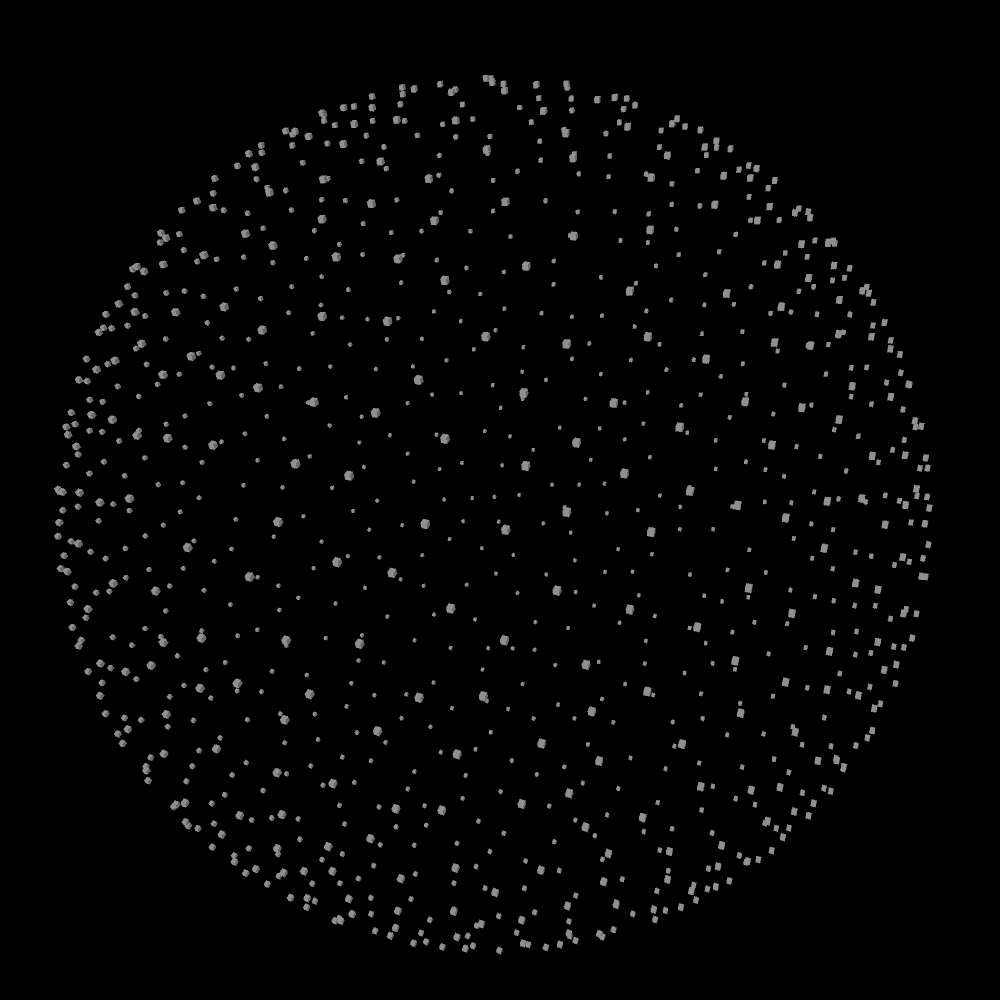Next I dug holes by removing anchors within certain distance to 14 centers on the sphere. 6 of these centers are easy to determine (as they have axis aligned directions), while for the other 8 diagonal ones, I numerically found the elevation angle to be 0.61548 radians.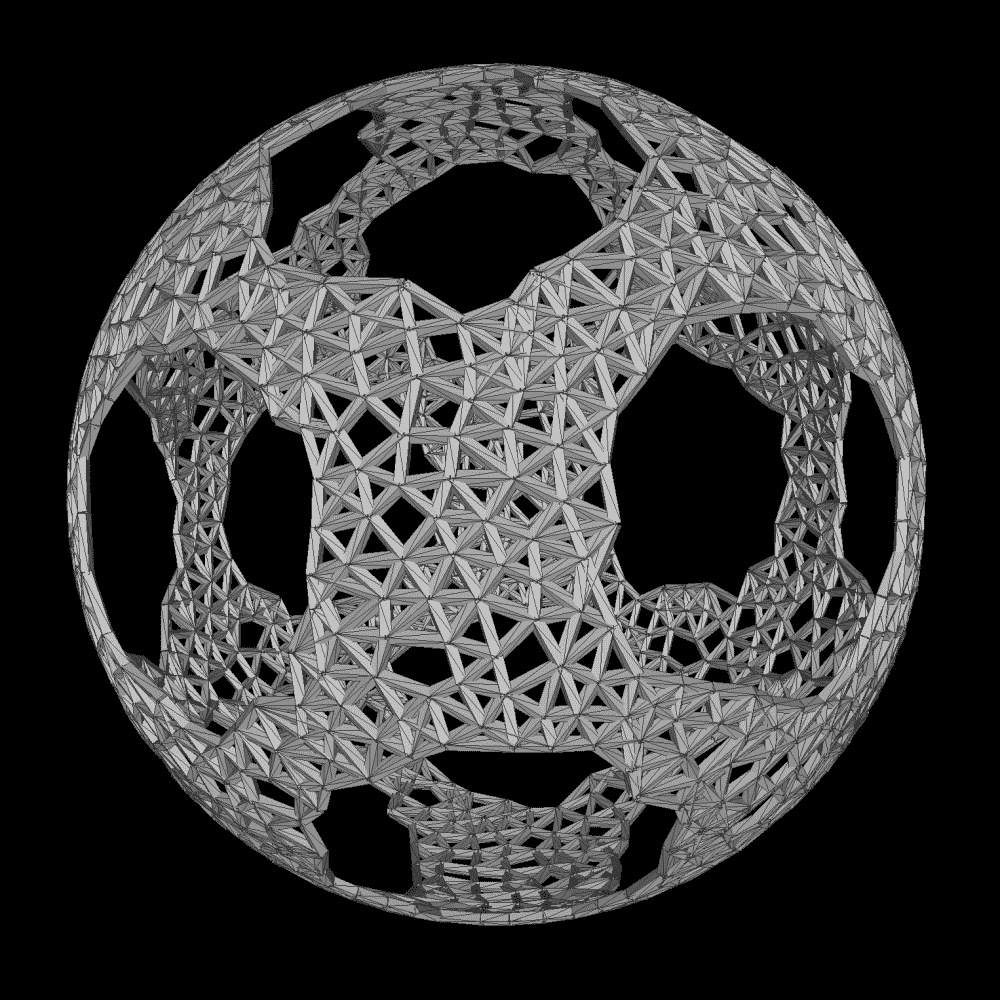Then I grew vines by each time starting with an arbitrary unvisited vertex, moving to neighbors iteratively and recording the path taken. Repeat until all vertices are visited. Initially I allowed vines to cross and overlap each other, the only rule being turns cannot be too sharp; Later I changed the rules, so that vines may not cross each other, and smoother turns are preferred but not mandatory, which I found to produce more aesthetically pleasing results. In retrospect, the method is basically a version of a maze creation algorithm.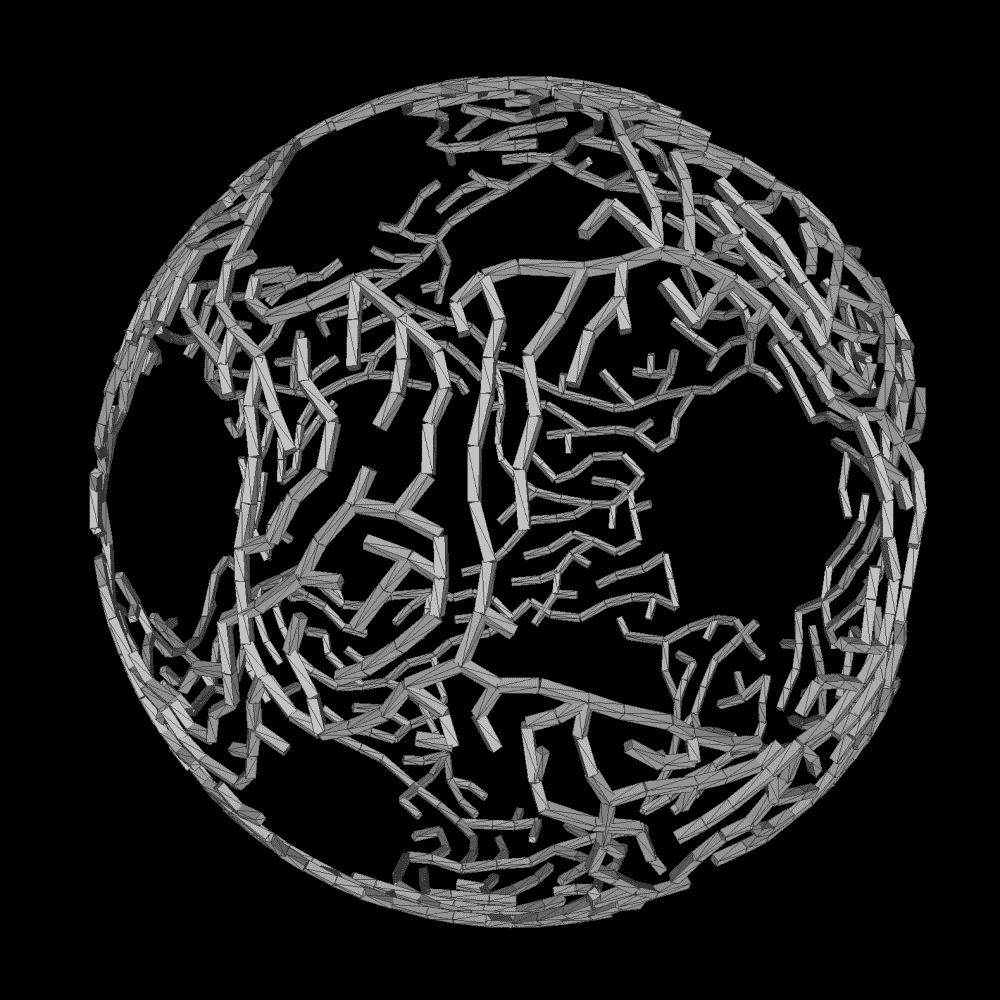After that I used 3D splines to smoothen the vine paths. Nowadays I prefer catmull-roms to beziers since they pass through all the control points.

Up till now I've been using simple stretched boxes to mark the vertices and edges, so that I can confirm my algorithms are working. Now I wanted to generate proper geometry for the vines. Since each vertex is on the unit sphere, its normal equals its position. I rotate the normal along the axis formed by the edge connecting a vertex to next for 360° to create the cross section. I squashed the cross section in the direction of the normal, so it is elliptical. The points on cross sections are then winded to create the mesh. Note that this is actually not the “correct” way to create tube geometry: with sharper turns the thickness would not be consistent, but since my curves are quite smooth and the vines don't serve a mechanical function, the errors are negligible.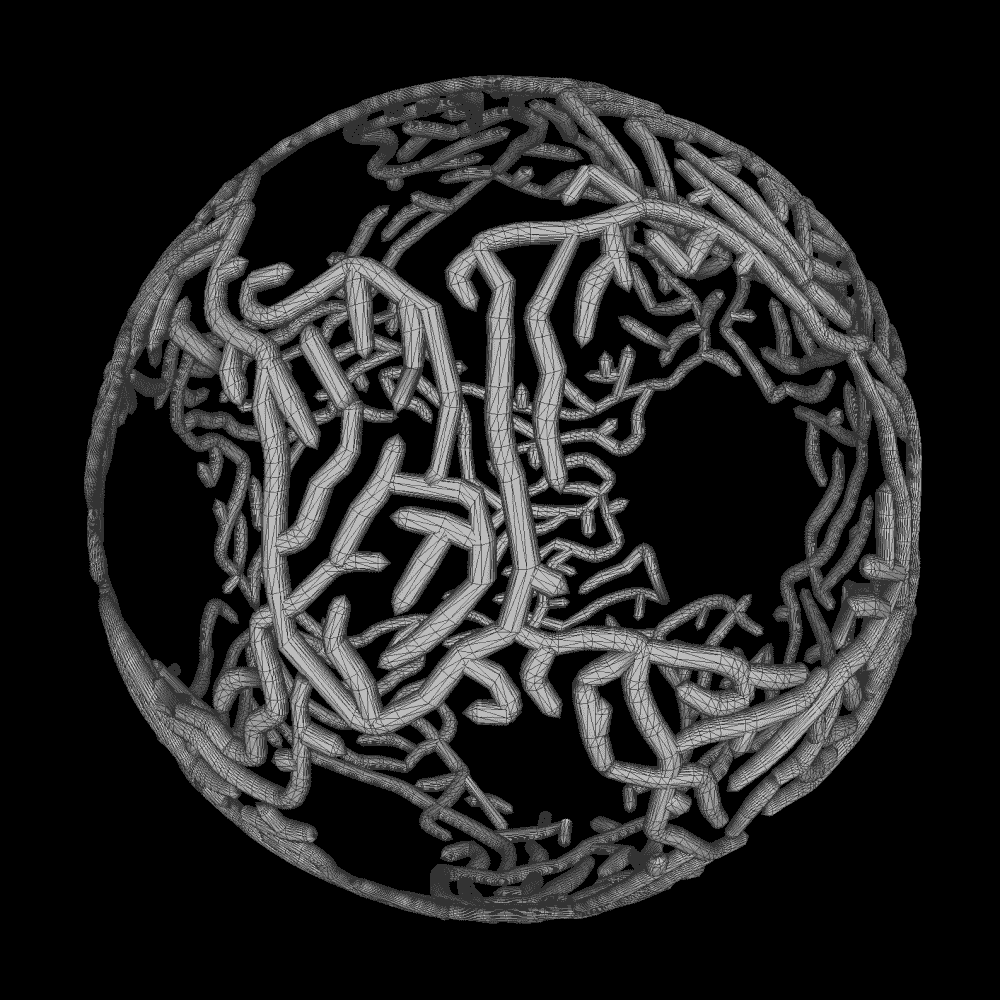The next step was to grow leaves on the vines. I enforce that every leaf keeps certain distance from each other, since otherwise the meshes clip through each other and don't look pretty. The function I used to model the general shape of a leaf is sin(pi*x^1.5). I then added bending along each of the X, Y and Z axes to better model the soft shape, before shifting the points with perlin noise to give it a more organic look. Again I winded together the sample points to create the leaf mesh.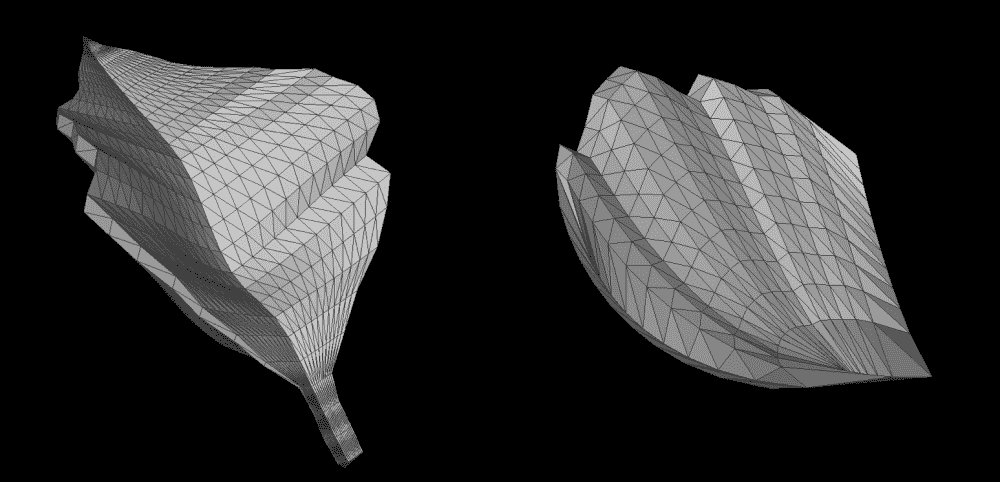To generate the flowers, I first wrote a function to generate a single petal, which is not unlike generating a leaf, except that the curves are modelled with different functions. Then, I placed petals of decreasing size around concentric circles to create a flower. Rotation, sizes and shapes were randomized to create natural look. Finally I added some little spheres and sticks as stamen -- very lazy, but looked good.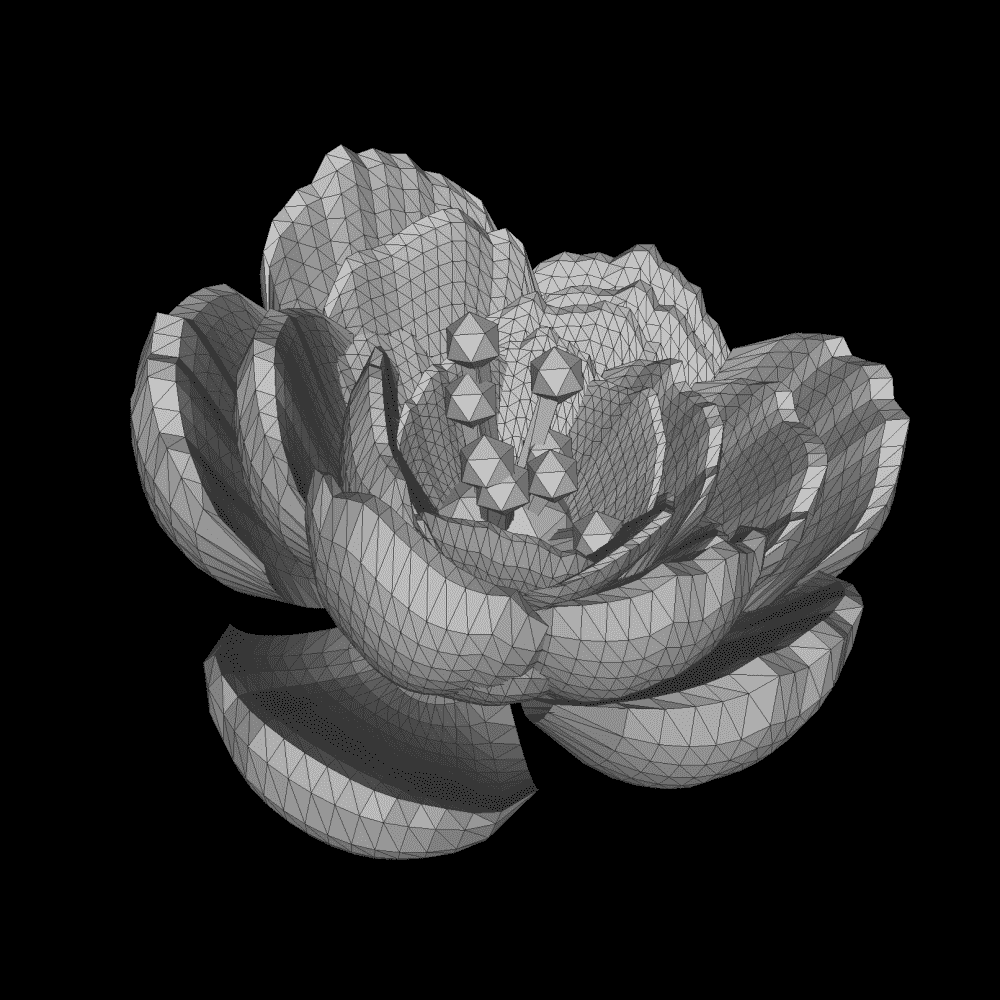I don't really know what kind of plant I'm generating. Does there exist a type of flower in nature that has those kind of vines and leaves?

As the final step to the outermost sphere, I decided to draw the edges between all adjacent anchors. These would help physically hold all the vines together so the sphere won't fall apart; Besides they also look nice.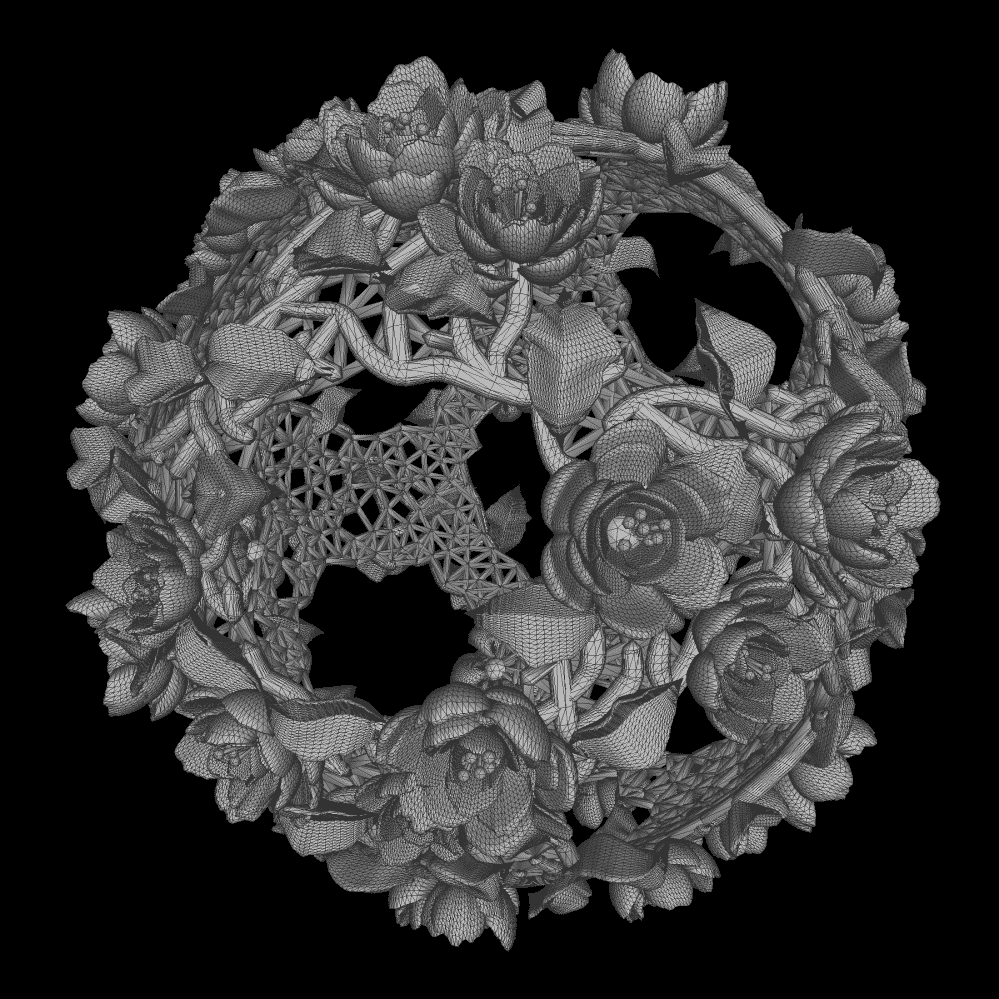For the inner spheres, I decided to use icospheres. Since the outer sphere had a very organic and chaotic look, the orderly patterns of icospheres should provide nice contrast. I also dug 14 holes on each of them with the same method as before, and connected adjacent edges with thick sticks with elliptical cross-section to create the surface. Finally a “disk” is fitted to each hole to give it a clean finish.Traditionally craftsmen of puzzle balls boasted the number of layers, some even reaching more than 20. I don't have this obsession and thought 4 was cool enough, at least for my first 3D print project.

## Generating the Texture

Since the stratasys J55 3D printer can print full color, I wanted to generate a texture map for my ball to utilize that advantage. My idea was to bake ambient occlusion (AO) to accentuate the forms in the geometry, like how some old sculptures look better when creases and crevasses turn brown. I didn't want to make red flowers, green leaves, etc. since that could very easily look ugly, especially when I'm not familiar with the color accuracy of the printer. So baked AO is the only safe approach.

I tried to bake it in blender but my mesh was too crazy, and blender either crashed, hanged, or output nonsensical results despite my multiple attempts. So much time was wasted that I was tempted to implement AO myself (its principles are not that complex at all). Just in time, I discovered that meshlab, the software I've been using to preview my STL files all this time, actually has AO feature built in. It took meshlab 2 seconds to generate a UV and another 2 seconds to bake the AO. I used “Trivial Per-Triangle” for the UV, so meshlab doesn't make any attempt at optimizing it, but for 3D printing the file size is not a great concern, and I simply specify 8192x8192 as the texture size to preserve all details. Meshlab's AO is “Per-Vertex” not per pixel, but the quality looks quite decent, I guess since there're already many vertices in my model.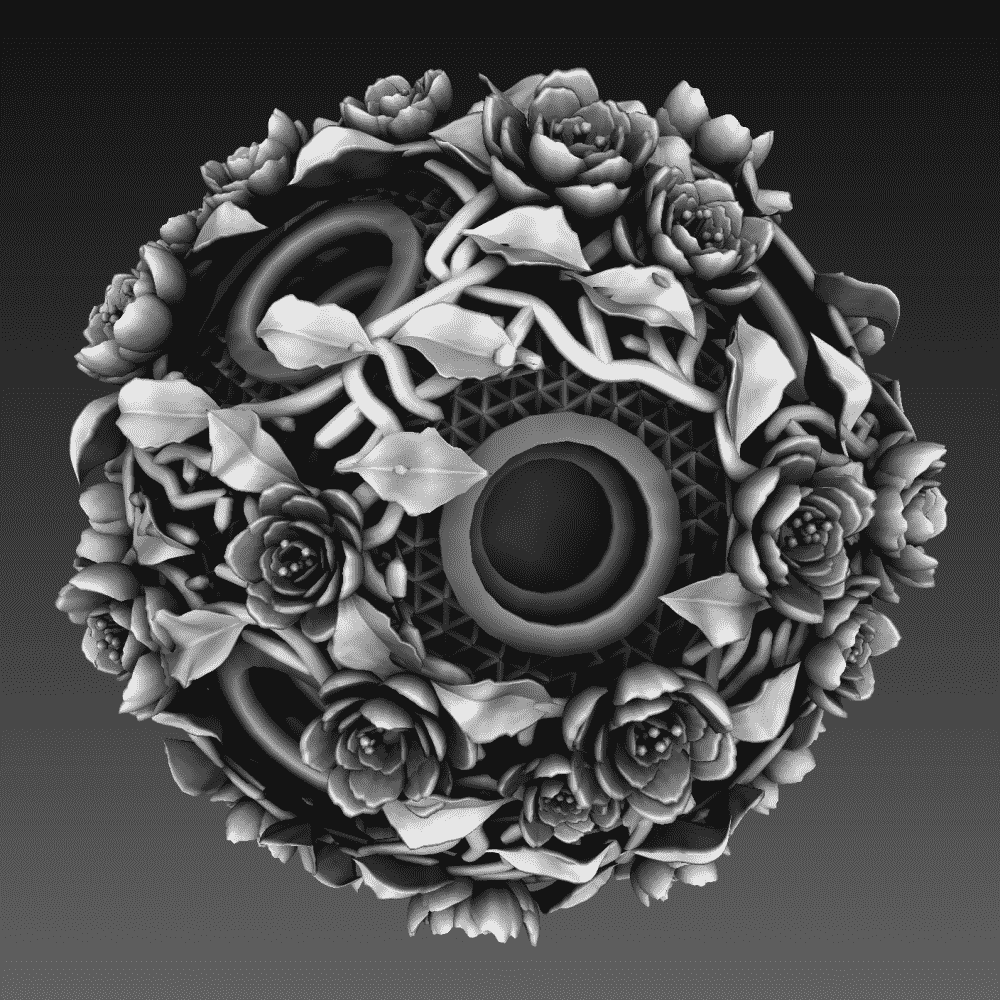After obtaining the AO, I wanted to tint the grayscale to a warm shade, with highlights toward the yellow end and shadows toward the red end. I used a simple python OpenCV script to achieve it in no time:

import numpy as np;
import cv2;

t = im.astype(np.float32)/255;

a = np.ones(im.shape,np.float32);
b = np.ones(im.shape,np.float32);

a[:,:,2] *= 0.27
a[:,:,1] *= 0.17
a[:,:,0] *= 0.1

b[:,:,2] *= 1.0
b[:,:,1] *= 1.0
b[:,:,0] *= 0.9

c = np.multiply(a,(1-t)) + np.multiply(b,t);

cv2.imwrite('out_tex.png',(c*255).astype(np.uint8));


I made a nice rendering of the finished model with blender, so I can stare at it narcissistically before the real model gets printed: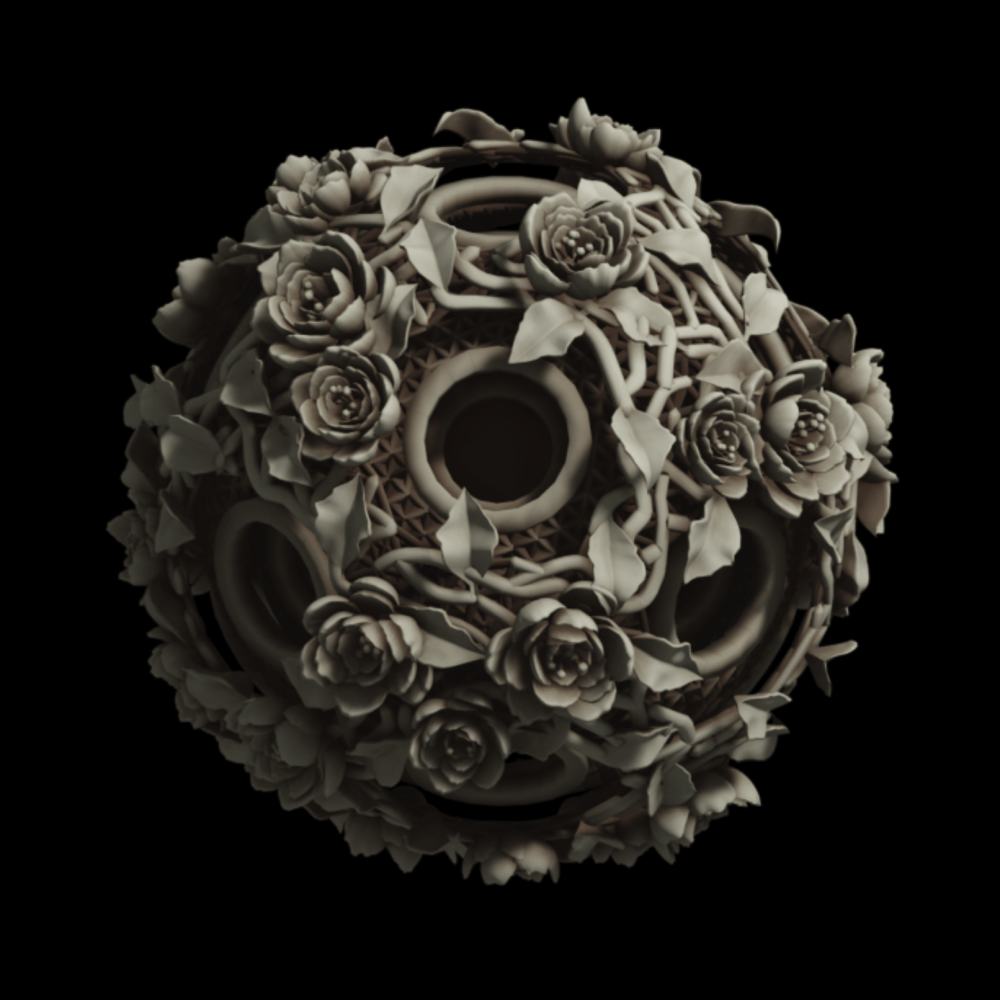Overall I'm quite happy with my design. Time-wise, I did some initial tests on Wednesday night, wrote the main part on Thursday between classes, and stayed up late Thursday night to submit the finished files on Friday morning in time for the 3pm batch on stratasys J55.

I could think of some improvements to the algorithms, though that would probably take much longer to code. For example, if the flowers and leaves were sculpted by a sculptor/craftsman, they would probably slop more over the surface underneath, like cheese in a hamburger; since my algorithms are additive not subtractive, mine look more like separate objects attached on top, they also clip through each other sometimes which is undesirable; perhaps some softbody simulation could be the solution.

## Printing

Since I'm planning to use J55 for my very delicate and multi-color design, my printing process simply involves sending my file to shop admin Tom, the only person authorized to touch the O(\$100k) machine.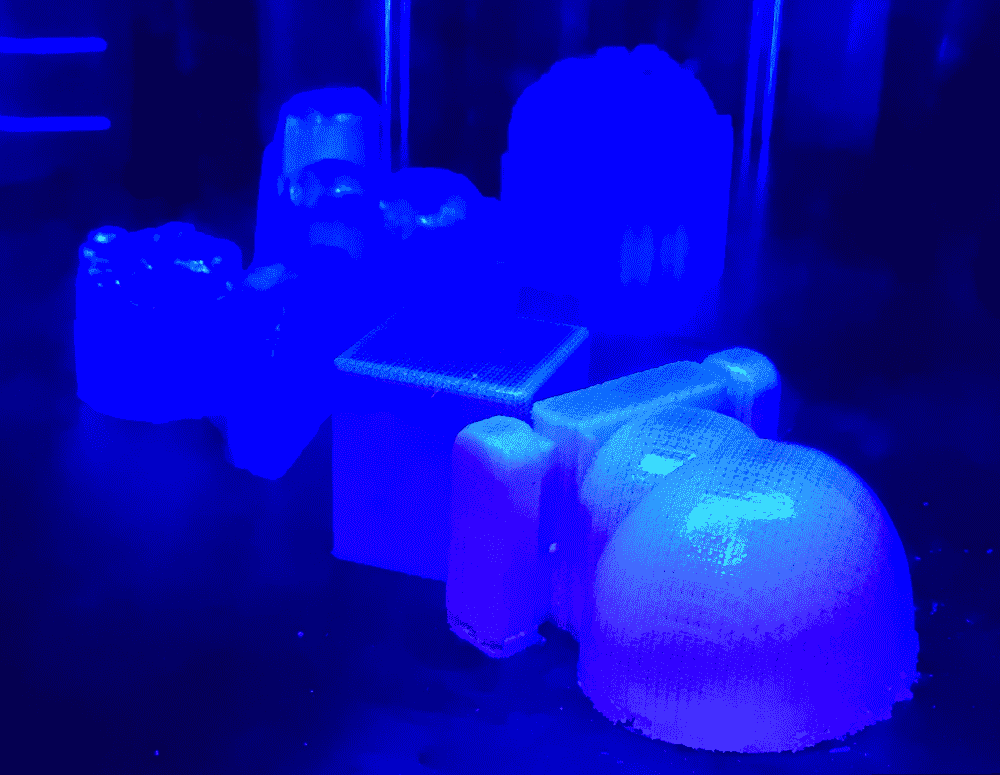I took a sneak peek at the finished batch on Saturday, before learning how to post-process it on Monday. (In the image above, mine is probably the furthest one in the background).

## Water-Gunning

On Monday Tom taught me how to use a really powerful water gun to clean off the support material from the 3D print. The gun was so powerful, that I had to hold my object really firmly, otherwise it would get blasted away!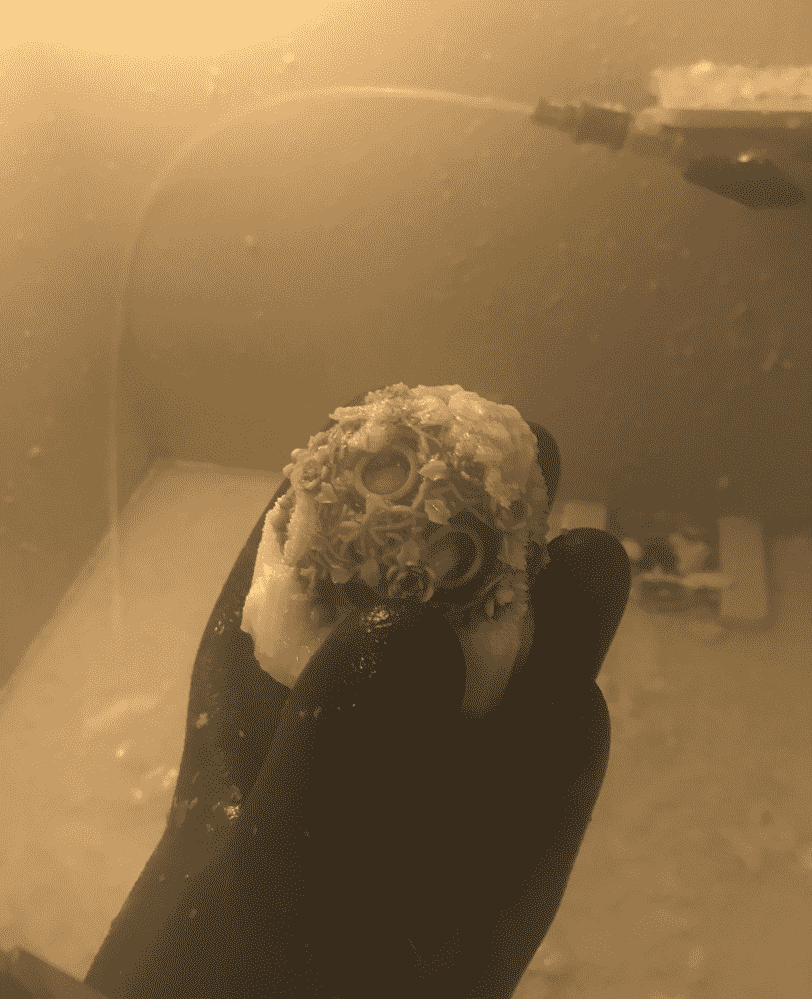Rather tragically, the overly powerful water gun blasted off a small piece of my ball's outer shell. I was so traumatized that I decided to stop blasting immediately. There were still some white goo in the crevasses but I decided they look like snow and aren't that ugly.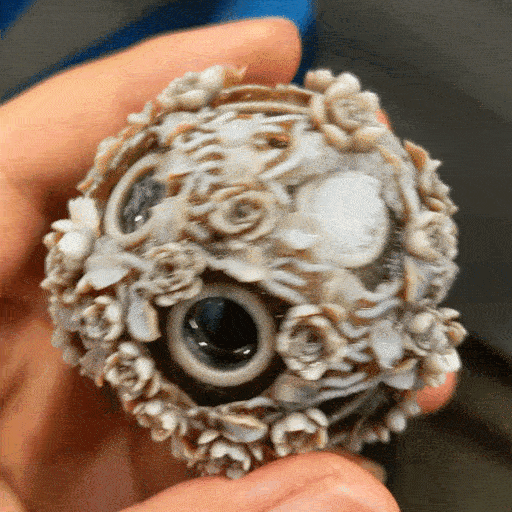## Ear-Picking

I stole some toothpicks at lunch time because I figured that besides goo in teeth, they might also pretty good at picking the goo in my print. Turned out my speculation was correct; so I sat down and took my time to clean up my print with one.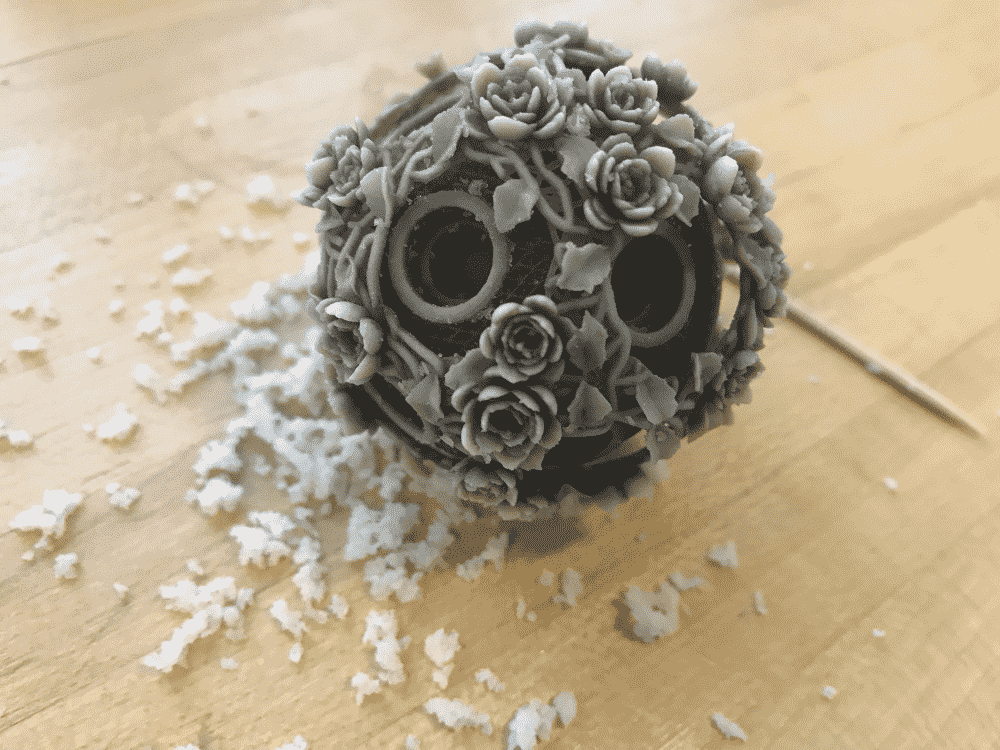The process was not unlike picking one's ear. In fact, the experience is exactly the same: the touch of the material, the skills required, the satisfaction after removing a large lump, etc. Only difference is that instead of picking two ears, I get to pick so many ears, like I'm picking ears for the whole class.

Initially, I didn't have high hopes that I could get the layers separated and move them independently (like how a Chinese puzzle ball should behave), especially after I learned that the support material were to be cleaned of with a water gun (and not with magical potion that miraculously melts away the bits I don't want, like I initially imagined). However, now equipped with a toothpick and superhuman patience, this becomes yet possible.

So I was just picking and picking, and all of a sudden the outer shell starts moving! I haven't removed all the support material in-between yet, but that was enough for the inner sphere to break free on its own. I was so excited that my hand becomes too shaky to continue working.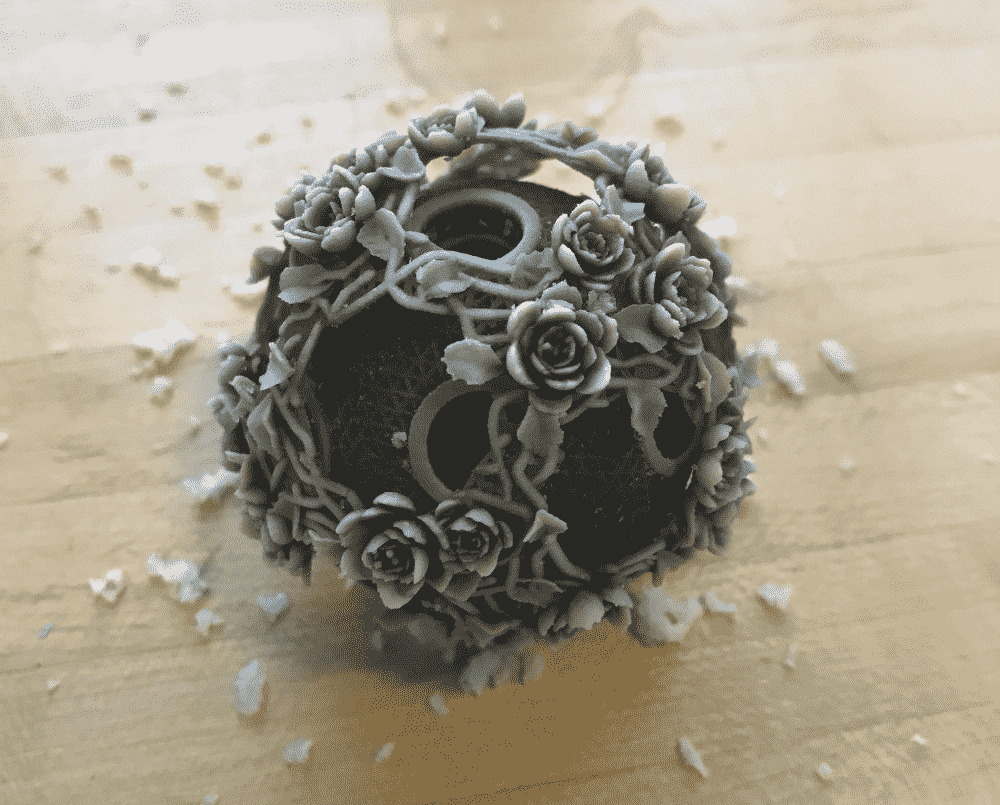Soon I cleaned up all the material between the two spheres. And although the inner ball doesn't rotate too smoothly due to all the sculptured surfaces, I thought it was already super cool to get the ball-inside-a-ball effect working at all. The three inner spheres I wasn't able to separate yet; I think they're too deep inside and I would need a more specialized tool. I heard the real Chinese puzzle ball carvers use a L-shaped tool -- maybe I can make one of those by bending some metal.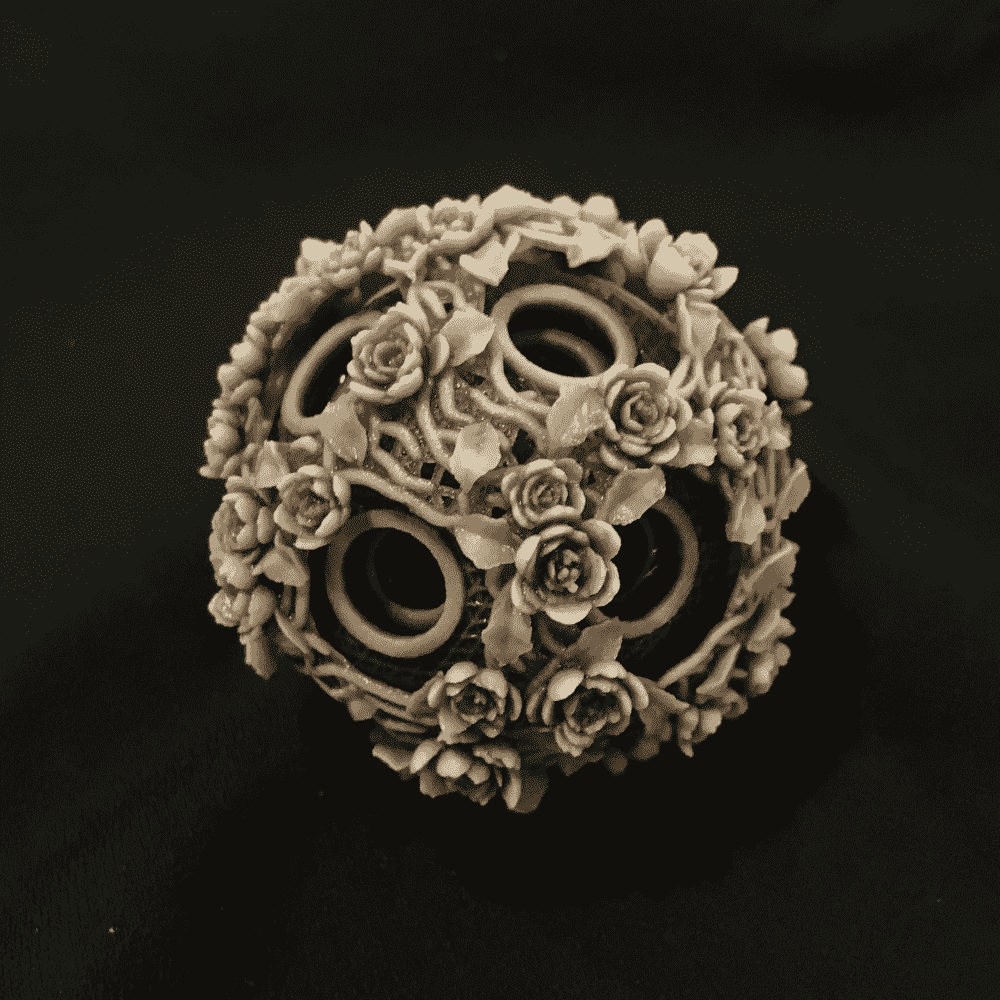Overall I'm happy with how the print turned out. The amazing J55 captured (almost) all the little details, even the stamen in the flowers, despite the size of the sphere being smaller than an egg. The ambient occlusion worked fairly well (it's hard to say how much better, since I haven't printed one without AO). The only regret is that a small part was blasted off by water gun -- but hey even those ancient artifacts usually end up breaking one part or another! If I were to print this design again, I would probably thicken the outermost shell (to prevent breaking), and reduce the number of layers to two or three (to simplify clean up), or, perhaps I could just print bigger!

You can find the online demo here, where you can generate your own spheres and download as STL to 3D print.